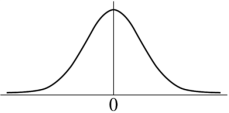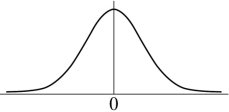• FAQ
• Contact/ Homework Answers / Statistics / For questions (6) and (7), shade the approximate area that
Not my Question
Flag Content

# Question

For questions (6) and (7), shade the approximate area that would represent the p-value for the alternative hypothesis and z-score, and then calculate the p-value.  Round to the nearest thousandth.

6. The alternative hypothesis is a right-tailed with a z-score = 0.217. The alternative hypothesis is a two-tailed with a z-score =−1.888. Two different students conduct a coin flipping experiment with a lefttailed alternative.  The obtain the following test statistics:

Student #1:  z =−2.05

Student #2:  z =−1.28

Which of the test statistics has a smaller p-value and why?

9. List and briefly summarize the essential ingredients of the hypothesis test.

10. Suppose the following is to be tested:  H0 : p= 0.4 and Ha : p0.4.  Calculate the observed z test statistic for the following sample data:  n= 80 and 25 test subjects have the characteristic of interest.  Round to the nearest thousandth.

## Solution 5 (1 Ratings )

Solved
Statistics 7 Months Ago 10 Views